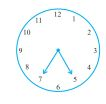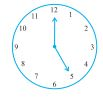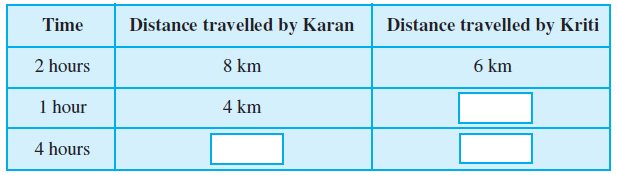## Filters

Sort by :
Q

Try to draw rough sketches of

(a) a scalene acute angled triangle.

(b) an obtuse angled isosceles triangle.

(a) a scalene acute angled triangle. :  Scalene : All side of different length Acute angled : All angles less than    (b) an obtuse angled isosceles triangle Isosceles traingle: Only two sides are of equal length Obtuse angled : At least one angle greater than

What does the angle made by the hour hand of the clock look like when it moves from 5 to 7. Is the angle moved more than 1                   right angle?No, the angle is not more than than 1 right angle.  For each hour, angle made =  Therefore, when the hour hand moves from 5 to 7, the angle made =

The hour hand of a clock moves from 12 to 5. Is the revolution of the hour hand more than 1 right angle?Yes, the revolution of the hour hand is more than 1 right angle. For each hour, angle made =  Therefore, when the hour hand moves from 12 to 5, the angle made =

Draw five other situations of one-fourth, half and three-fourth revolution on a clock.

(a) One fourth revolution: From (b) Half revolution: From (c) Three fourth revolution: From (d) Three fourth revolution: From (e) Half fourth revolution: From

What is the angle name for one-fourth revolution?

One-fourth revolution =  The angle name for one-fourth revolution is "Right Angle"

What is the angle name for half a revolution?

Half a revolution =  The angle name for half a revolution is "Straight Angle".

Read the table and fill in the boxes.distance travelled by kriti in 1 hour is 3km

distance travelled by karan in 4 hours is 16km

distance travelled by kriti in 4 hours is 12km

Check whether the given ratios are equal, i.e. they are in proportion.
If yes, then write them in the proper form.
1. 1 : 5 and 3 : 15
2. 2 : 9 and 18 : 81
3. 15 : 45 and 5 : 25
4. 4 : 12 and 9 : 27
5. ` 10 to ` 15 and 4 to 6

1. 1 : 5 and 3 : 15 So the ratios are in proportion 2. 2 : 9 and 18 : 81 So So the ratios are in proportion 3. 15 : 45 and 5 : 25 The given ratios are not equal, so they are not in proportion 4. 4 : 12 and 9 : 27 The given ratios are equal, so they are in proportion 5. ` 10 to ` 15 and 4 to 6 The given ratios are equal, so they are in proportion

Draw any rectangle and find the ratio of its length to its breadth.

Suppose a rectangle has length 10 cm and breadth of 7 cm then ratio of its length to its breadth=10:7

Find the ratio of number of doors and the number of windows in your
classroom.

If there are four windows and one door then the ratio of the number of doors and the number of windows =1:4

Find the number of students above twelve years of age in your class. Then, find the ratio of the number of students with age above twelve years and the remaining students.

suppose there are 40 students in the class and 5 students are above 12 years, then there are 40-5=35 students below or equal to 12 years. Then he ratio of the number of students with age above twelve years and the remaining students = 5:35=1:7

Find the ratio of number of desks and chairs in your classroom.

If there are 8 desk and 32 chairs then the ratio of number of desks and chairs is 8:32=1:4

Find the ratio of number of notebooks to the number of books in your bag.

If there are 3 notebooks and 4 books in the bag then the  ratio of the number of notebooks to the number of books =3:4

In a school, there were 73 holidays in one year. What is the ratio of the number of holidays
to the number of days in one year?

Number of holidays in a year = 73 Number of days in a year = 365   the ratio of the number of holidays to the number of days in one year= 1:5

Cost of a toffee is 50 paise and cost of a chocolate is rupees 10. Find the ratio of the cost of a toffee to the cost of a chocolate.

Cost of toffee = 50 paise cost of chocolate =  10 rupees 1rupee = 100 paise Therefore, 10rupee = 1000 paise  So, the cost of chocolate = 1000 paise  the ratio of the cost of toffee to the cost of chocolate=50:1000=1:20

Saurabh takes 15 minutes to reach school from his house and Sachin takes one hour to reach school
from his house. Find the ratio of the time taken by Saurabh to the time taken by Sachin.

Time taken by Saurabh = 15 minutes Time taken by Sachin= 1 hour = 60 minutes.  To find ratios we have to convert the given quantities to the same units. Here we are expressing both the quantities in minutes  the ratio of the time taken by Saurabh to the time taken by Sachin= 15:60=1:4

Ravi walks 6 km in an hour while Roshan walks 4 km in an hour What is the ratio of the distance covered by Ravi to the distance covered by Roshan?

The distance covered in one hour by Ravi = 6 Km   The distance covered in one hour by Roshan =  4 Km So the required ratio is 3:2

In a class, there are 20 boys and 40 girls. What is the ratio of the number of boys to the number of girls?

number of boys = 20 number of girls = 40 So the required ratio is 1:2

Q.2.    Find the area of any one door in your house.

suppose the length of door is 2m and breadth is 0.5 m  then the area of door =

Find the area of the floor of your classroom.

If floor length is 3m and breadth is 2m then
Exams
Articles
Questions